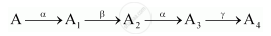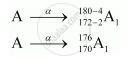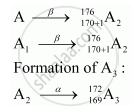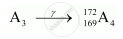Department of Pre-University Education, KarnatakaPUC Karnataka Science Class 12

# A Radioactive Nucleus ‘A’ Undergoes a Series of Decays According to the Following Scheme:The Mass Number and Atomic Number of a Are 180 and 72 Respectively. What Are These Numbers for A4? - Physics

A radioactive nucleus ‘A’ undergoes a series of decays according to the following scheme:The mass number and atomic number of A are 180 and 72 respectively. What are these numbers for A4?

#### Solution

A has mass number as 180 and atomic number 172.

Formation of A1 by α-decay:Formation of A2 by β decay:Formation of A4:

In r-decay, mass number and atomic number remain the same.

Thus,Mass number of A4 = 172

Atomic number of A4­ = 169

Is there an error in this question or solution?